# Algebra 1 : How to graph a two-step inequality

## Example Questions

### Example Question #32 : Graphing

Which graph depicts the following inequality?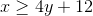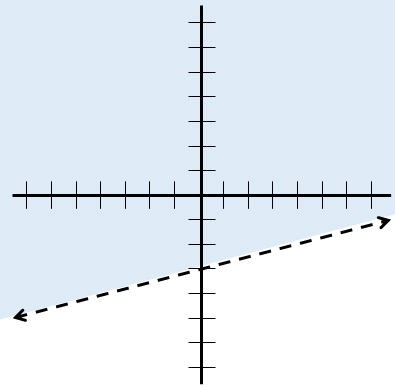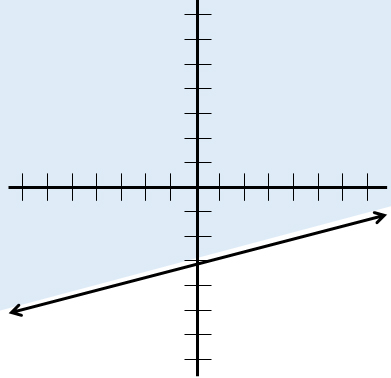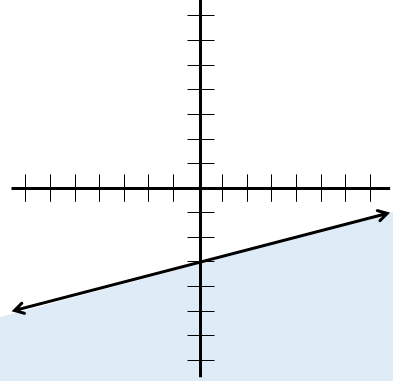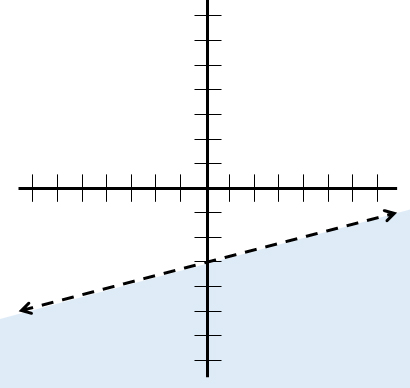No real solution.Explanation:

Let's put the inequality in slope-intercept form to make it easier to graph: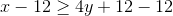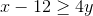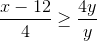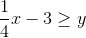The inequality is now in slope-intercept form. Graph a line with slopeand y-intercept.

Because the inequality sign is greater than or equal to, a solid line should be used.

Next, test a point. The origin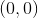is good choice. Determine if the following statement is true: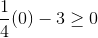The statement is false. Therefore, the section of the graph that does not contain the origin should be shaded.